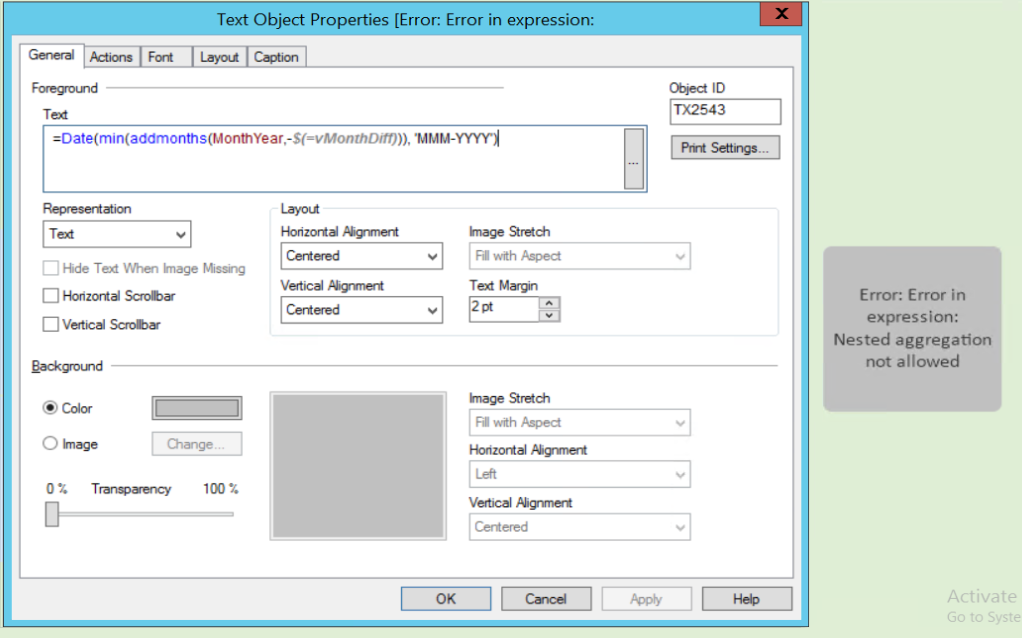New to QlikView

Discussion board where members can get started with QlikView.

Announcements
QlikWorld 2020: Join us May 11 - 14, 2020 in Phoenix, AZ. Register early and save \$400. Learn More
Contributor II

Subtract from AddMonths with variable

Hi,

I'm working with dates.

I have MonthYear ('MMM-YYYY').In this example I've choose from apr-2016 to jun-2016.

I've created a function MonthDiff that gives me the number of months selected, in this case, 3.

I want to get the previous "MonthDiff" months from the date I've selected.

Example:

If I choose from apr-2016 to jun-2016, so I want previous to be jan-2016 to mar-2016.

Or if I choose from jan-2016 to jun-2016, I want previous to be jul-2015 to dez-2015.

Going back to my MonthDiff = 3

I want to do the next expression to get the value jan-2016

But this is not working, it's giving me the error "Error: Error in expression: Nested aggregation no allowed"

How can I solve this problem and achieve what I want?

Is it possible?

Sílvia

1 Solution

Accepted SolutionsMVP

Re: Subtract from AddMonths with variable

9 RepliesMVP

Re: Subtract from AddMonths with variable

What does your \$(vMonthDiff) expand to ?

The expression itself looks fine, as long as it expands to an integer.MVP

Re: Subtract from AddMonths with variable

Have you created variable there,  does the variable  gives the 3  at this  situation.  Can you please let me know the value or string you are getting got that

Before develop something, think If placed (The Right information | To the right people | At the Right time | In the Right place | With the Right context)
Contributor III

Re: Subtract from AddMonths with variable

Hi Siliva,

exp 1 :SUM({<Year = {\$(=only(Year))}, Month = {"<=\$(=num(Month))"}>} [Sales Amount])

exp 2: SUM({<Year = {\$(=only(Year)-1)}, Month = {"<=\$(=num(Month))"}>} [Sales Amount])

if select year = 2016 month = nov

exp 1 shows sum of sales from jan to nov individually

exp2 shows previous year sum of sales from jan to nov  individually

you have month year as same column

sum( monthyear = {"<=\$(=(monthyear))"}[sales amount])

sum( monthyear = {"<=\$(=(monthyear)-1)"}[sales amount])

Regards

Hemanth

Contributor II

Re: Subtract from AddMonths with variable

Hi,

This is my MonthDiff Variable:

= Num(((year(max(MonthYear)) * 12) + month(max(MonthYear))) - (((year(min(MonthYear)) * 12) + month(min(MonthYear)))) + 1)

In a separated text object, is giving me the value 3MVP

Re: Subtract from AddMonths with variable

Your variable expression is being expanded to its text content and thus contains aggregation functions such as max which mean you are trying to nest aggregations which is not allowed as the error message says.

Try putting an equals sign before the variable to evaluate it to an integer

Also have a look at this Blog post, The Little Equals Sign

Contributor II

Re: Subtract from AddMonths with variable

Hi Bill,

I've tried what you've suggested, but is still not working.What am I doing wrong?

Contributor III

Re: Subtract from AddMonths with variable

Hi Siliva,

try this:

Dispaly2 : =Num(((year(max(Disbursement_MonthYear)) * 12) + month(max(Disbursement_MonthYear))) - (((year(min(Disbursement_MonthYear)) * 12) + month(min(Disbursement_MonthYear)))) + 1)

it the above expression it shows expression ok in text boxregards

HemanthMVP

Re: Subtract from AddMonths with variable

Thanks a lot, your suggestion works perfectly